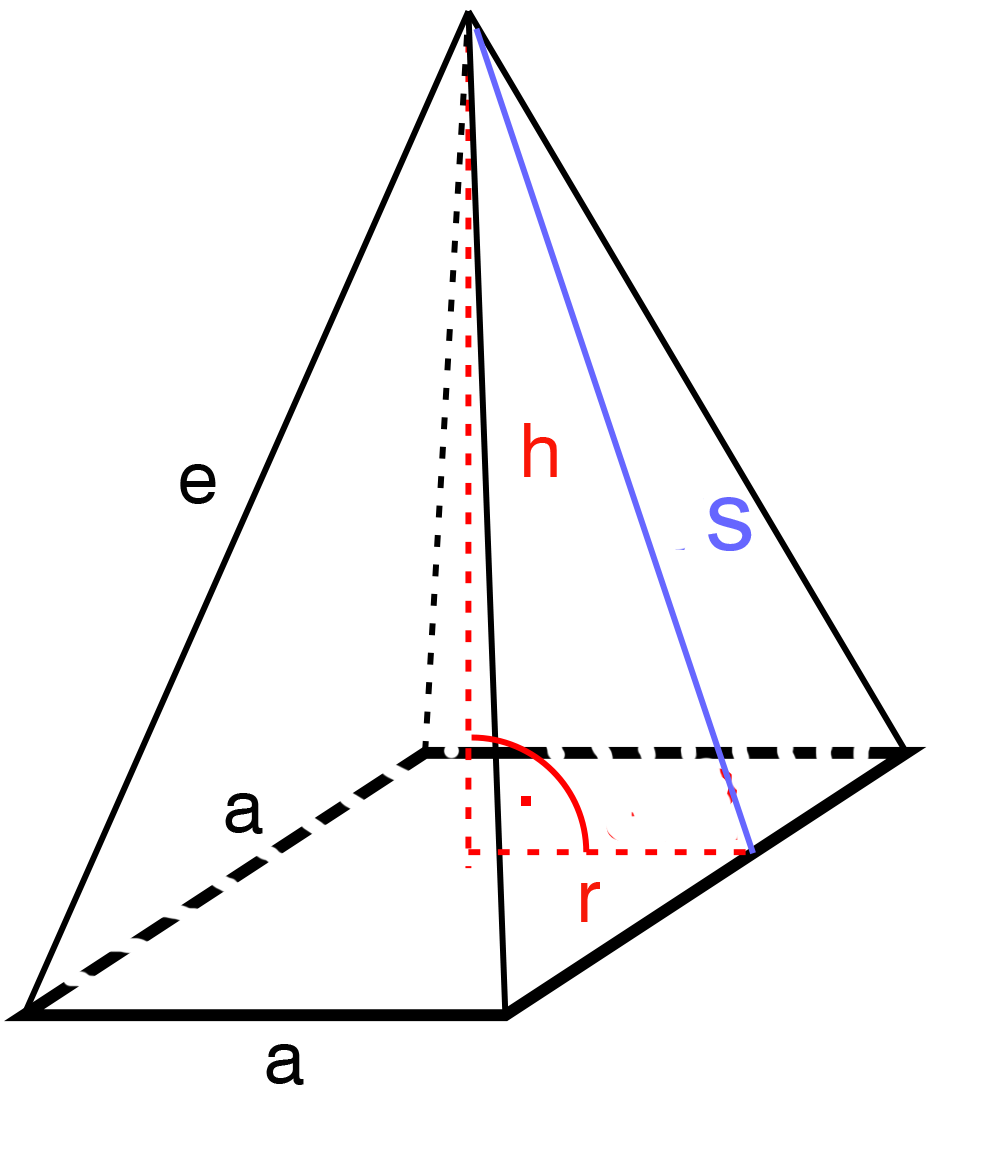﻿ Pyramid - square based: - on line calculation, formula - FORMIAX

# Pyramid (square based) surface and volumeS = SB+SL

SB = a*a

SL = 4*((a*s)/2)*4

V=(SB*v)/3

S = surface area

V = volume

a = side "a"

h = height

s = slant triangle height

SB = basement suface

SC = lateral surface

WHAT IS IT ?
Pyramid is a solid object where:
• The base is a polygon (a straight-sided flat shape)
• The sides are triangles which meet at the top (the apex).

CALCULATION:

Enter unit e.g.: inch

Enter side"a"

Enter height

Round to number of decimals places

YOU MIGHT BE INTERESTED: